# Bayesian Regression

greta and Stan go for a walk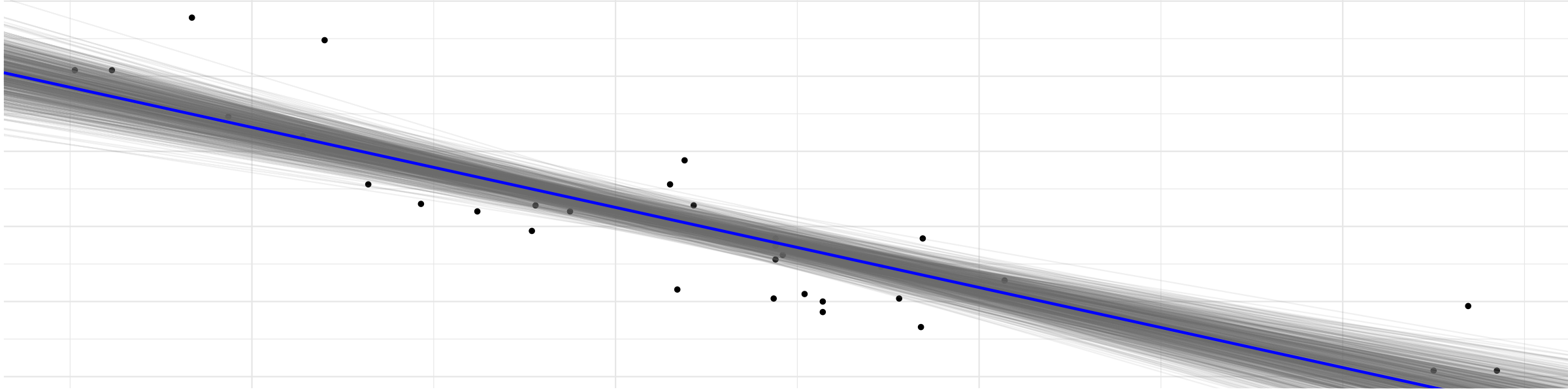I have been working on my Bayesian statistics skills recently. In particular, I have been reading David Robinson’s lovely Introduction to Empirical Bayes: Examples from Baseball Statistics and watching Rasmus Bååth’s delightful three-part Video Introduction to Bayesian Data Analysis, notable amongst other videos, courses, and textbooks. I have much yet to learn, but my past experience with statistics has taught me that I understand concepts most thoroughly by actually implementing them. Thus, this post is a neophyte’s pass at implementing Bayesian regression with some powerful (and complicated) tools. As such, it almost certainly contains some inaccuracies and statistical failings. (If you notice them, please let me know!) Nonetheless, I present it with the motivation that seeing variations in others’ implementations of code helps us see more options and pick better ones when writing our own.

## The example

What follows is two implementations of Bayesian linear regression with Stan and greta, two interfaces for building and evaluating Bayesian models. The example is adapted from the Stan (§9.1, p. 123 of the PDF) and greta docs.

It is a very simple linear regression of a single variable. Its frequentist equivalent would be

lm(mpg ~ wt, mtcars)
#>
#> Call:
#> lm(formula = mpg ~ wt, data = mtcars)
#>
#> Coefficients:
#> (Intercept)           wt
#>      37.285       -5.344

or visually,

library(ggplot2)
theme_set(hrbrthemes::theme_ipsum_rc())

ggplot(mtcars, aes(wt, mpg)) +
geom_point() +
geom_smooth(method = "lm")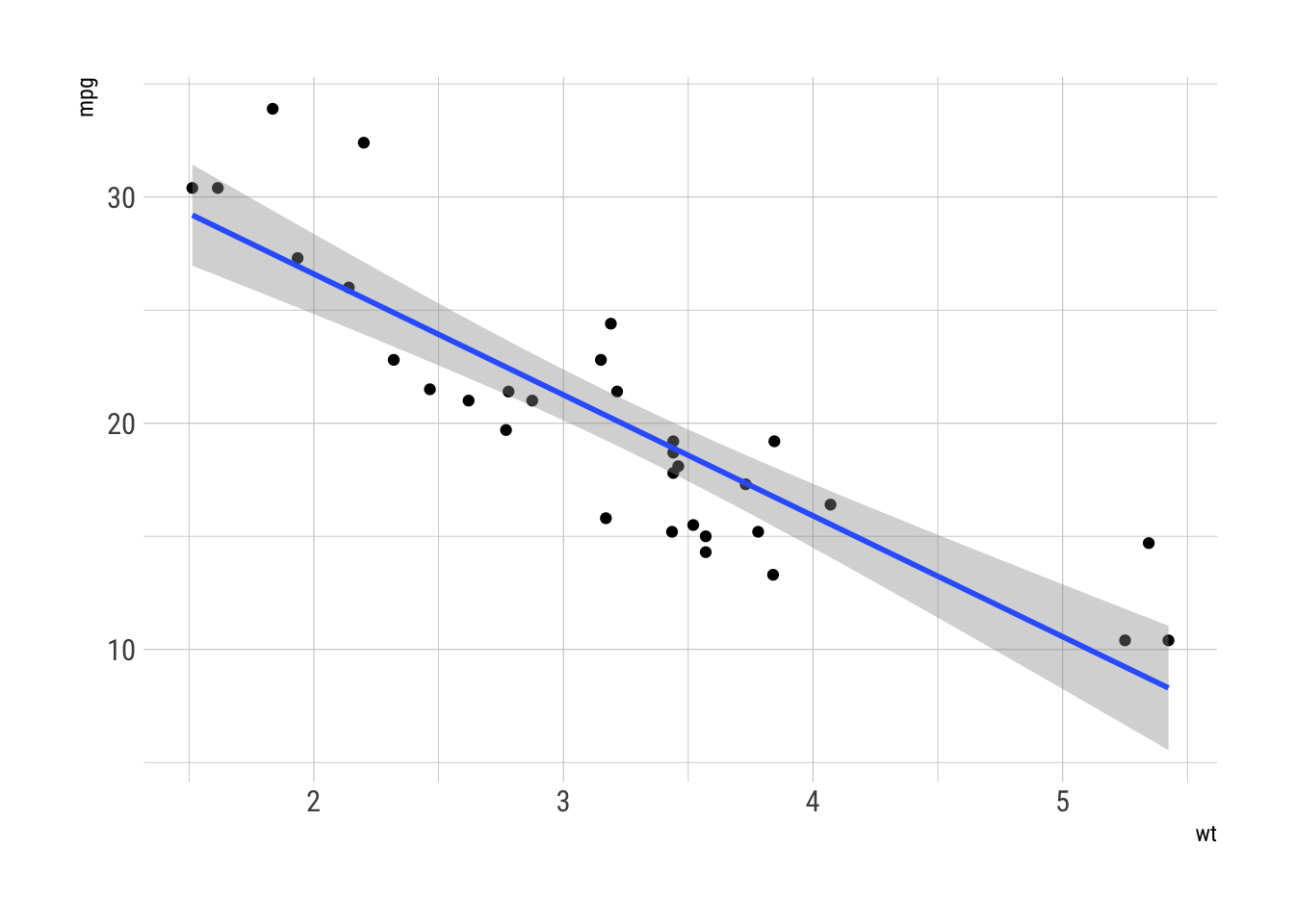## The tools

### Stan

Stan is a DSL for implementing Bayesian models. The model is composed in Stan, and then compiled and called from an interface in another language—in R, the rstan package. The Stan code compiles to C++, and rstan makes parallelization across chains simple.

It has its own shirts.

### greta

greta is an R package by Nick Golding that implements MCMC sampling in TensorFlow, and can thus run on GPUs and scale well, provided the appropriate hardware and drivers. It is built on top of RStudio’s tensorflow package.

It does not have shirts, as far as I am aware, but does have a nice logo that would make a handsome hex sticker.

## The code

### Stan

The Stan model has to be composed in, well, Stan. This can be in a separate file or a string, but I find the former usually too disconnected from the rest of the code and the latter a magnet for typos. The better solution in my attempts thus far is to implement the model in an RMarkdown code chunk with an output.var parameter set. When the chunk is run, the model is compiled and assigned to an R object with the supplied name. Code highlighting, completion, and so on work as usual.

Stan is declarative imperative1, with chunks to define input data, parameters, the model, and more as necessary. Keeping it very simple, we will supply

• n, a number of observations,
• x, our single feature (a vector of length n), and
• y, our output (another vector of length n).

The model will calculate

• beta0, the y intercept,
• beta1, the slope, and
• sigma, the standard deviation of y.

While we could supply informative priors by adding lines like beta0 = normal(30, 2);, we will instead leave it blank, so Stan will use a uniform prior, determining a plausible range by running lots of warm-up iterations.

Our model is just a line. We assume our outputs are normally distributed, with a mean defined by the line, and a standard deviation parameterized by sigma.

All together, and set to output to an R variable called model_stan:

data {
int<lower=0> n;
vector[n] x;
vector[n] y;
}

parameters {
real beta0;
real beta1;
real<lower=0> sigma;
}

model {
y ~ normal(beta0 + beta1*x, sigma);
}

Compiling takes about 40 seconds on my computer. It also produces 15 warnings that

warning: pragma diagnostic pop could not pop, no matching push [-Wunknown-pragmas] #pragma clang diagnostic pop

but apparently they’re harmless, so I set message=FALSE.

To run the model, we just load rstan and call sampling on the compiled model, supplying the data. I set the algorithm to Hamiltonian Monte Carlo and the number of chains to 1 so as to compare more equally with greta.

library(rstan)

draws_stan <- sampling(model_stan,
data = list(n = nrow(mtcars),
x = mtcars$wt, y = mtcars$mpg),
seed = 47,
algorithm = "HMC",
chains = 1)
#>
#> SAMPLING FOR MODEL 'mtcars' NOW (CHAIN 1).
#>
#> Gradient evaluation took 2.3e-05 seconds
#> 1000 transitions using 10 leapfrog steps per transition would take 0.23 seconds.
#>
#>
#> Iteration:    1 / 2000 [  0%]  (Warmup)
#> Iteration:  200 / 2000 [ 10%]  (Warmup)
#> Iteration:  400 / 2000 [ 20%]  (Warmup)
#> Iteration:  600 / 2000 [ 30%]  (Warmup)
#> Iteration:  800 / 2000 [ 40%]  (Warmup)
#> Iteration: 1000 / 2000 [ 50%]  (Warmup)
#> Iteration: 1001 / 2000 [ 50%]  (Sampling)
#> Iteration: 1200 / 2000 [ 60%]  (Sampling)
#> Iteration: 1400 / 2000 [ 70%]  (Sampling)
#> Iteration: 1600 / 2000 [ 80%]  (Sampling)
#> Iteration: 1800 / 2000 [ 90%]  (Sampling)
#> Iteration: 2000 / 2000 [100%]  (Sampling)
#>
#>  Elapsed Time: 0.23787 seconds (Warm-up)
#>                0.034739 seconds (Sampling)
#>                0.272609 seconds (Total)

draws_stan
#> Inference for Stan model: mtcars.
#> 1 chains, each with iter=2000; warmup=1000; thin=1;
#> post-warmup draws per chain=1000, total post-warmup draws=1000.
#>
#>         mean se_mean   sd   2.5%    25%    50%    75%  97.5% n_eff Rhat
#> beta0  37.02    0.08 1.53  34.08  36.02  36.96  37.99  40.10   402 1.00
#> beta1  -5.27    0.02 0.46  -6.15  -5.57  -5.25  -4.96  -4.43   389 1.00
#> sigma   3.10    0.01 0.36   2.46   2.86   3.08   3.31   3.94  1000 1.00
#> lp__  -51.08    0.10 1.28 -54.51 -51.61 -50.73 -50.17 -49.65   179 1.01
#>
#> Samples were drawn using HMC(diag_e) at Fri May  4 19:52:44 2018.
#> For each parameter, n_eff is a crude measure of effective sample size,
#> and Rhat is the potential scale reduction factor on split chains (at
#> convergence, Rhat=1).

Since this is a very small model, it runs nearly instantaneously (0.26 seconds). The print methods of the call and resulting model are nicely informative. It looks good, but let’s check the diagnostic plots:

plot(draws_stan)
#> ci_level: 0.8 (80% intervals)
#> outer_level: 0.95 (95% intervals)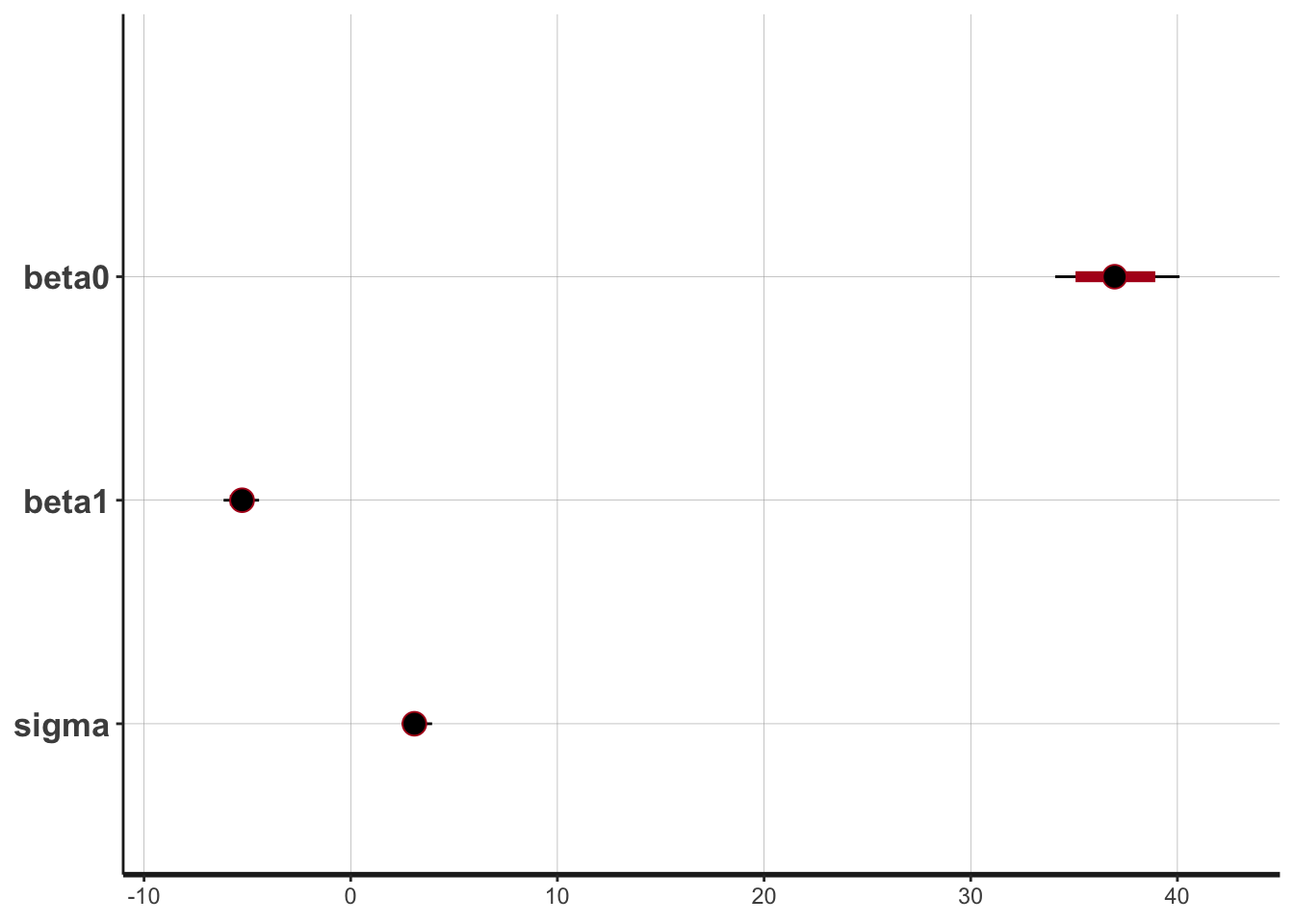traceplot(draws_stan)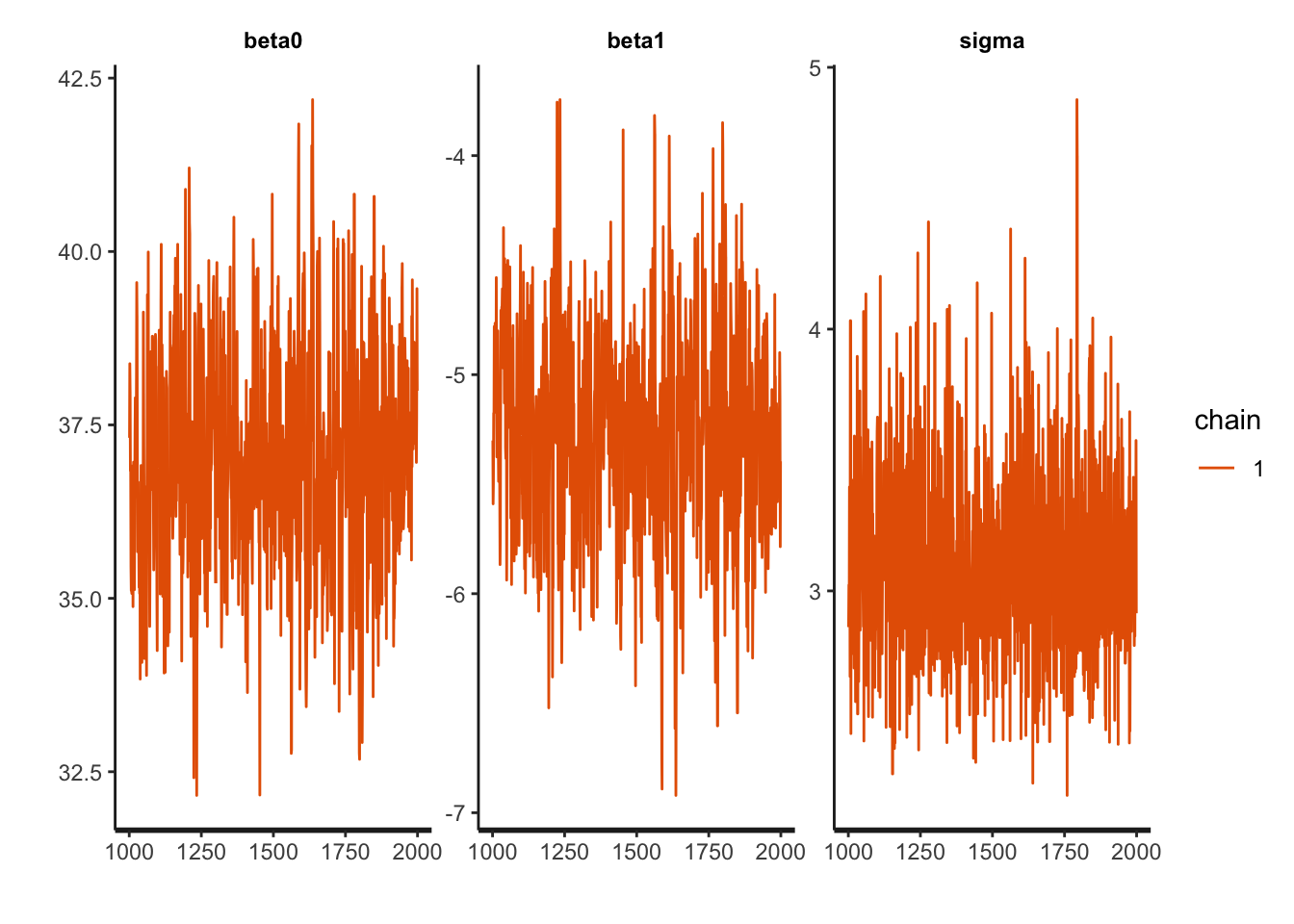The intercept is moving more than the slope or standard deviation, which is reasonable considering the dimensions. The data is not totally linear (it could be better fit with a quadratic function), but the traceplots are fuzzy caterpillars, so it seems all is well.

Let’s extract the data and plot:

draws_stan_df <- as.data.frame(draws_stan)

ggplot(draws_stan_df, aes(beta0, beta1, color = sigma)) +
geom_point(alpha = 0.3) +
geom_density2d(color = "gray30") +
scale_color_viridis_c(option = "plasma") +
labs(title = "Stan parameter space")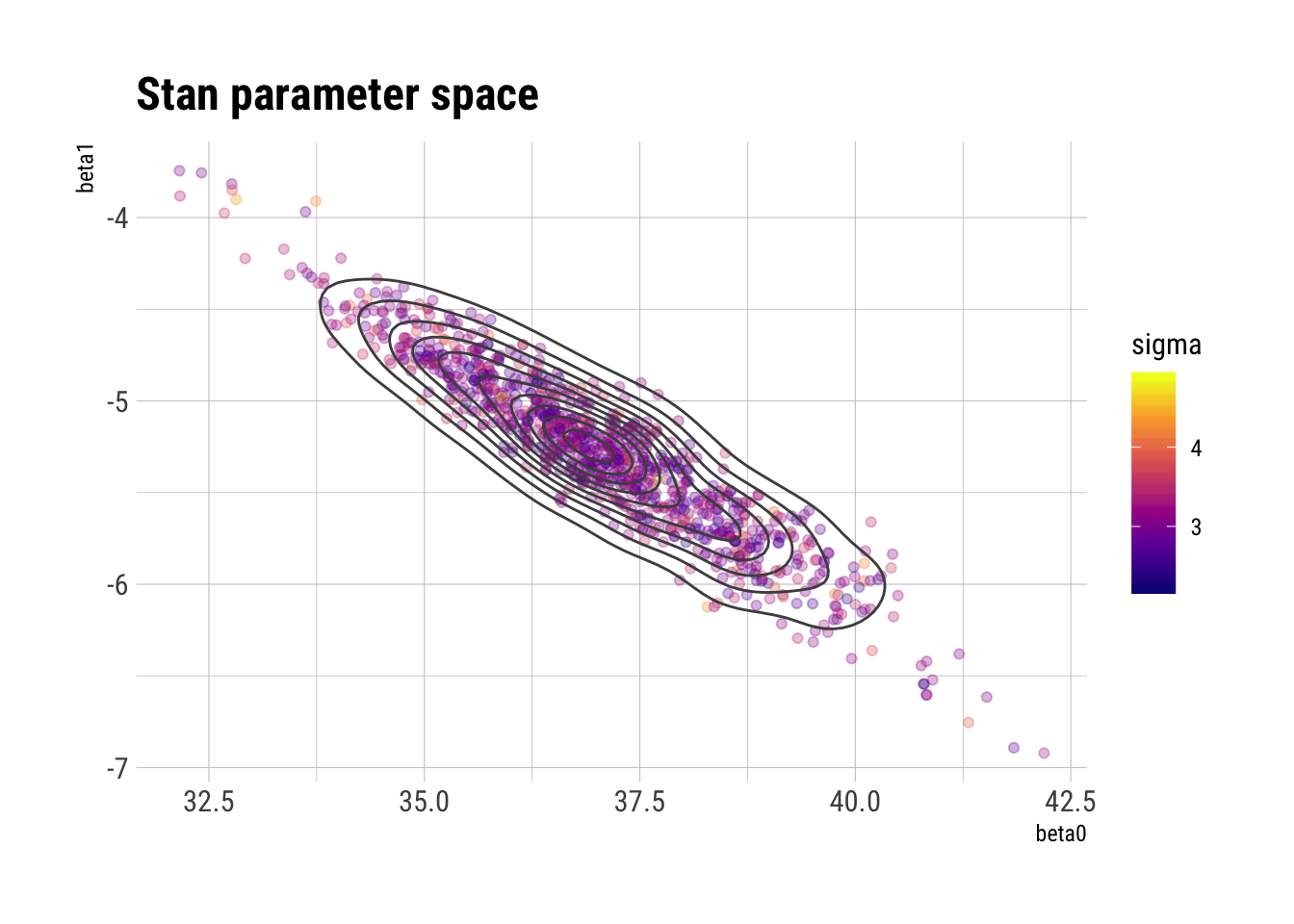ggplot(mtcars, aes(wt, mpg)) +
geom_point() +
geom_abline(aes(intercept = beta0, slope = beta1), draws_stan_df,
alpha = 0.1, color = "gray50") +
geom_abline(slope = mean(draws_stan_df$beta1), intercept = mean(draws_stan_df$beta0),
color = "blue", size = 1) +
labs(title = "Bayesian regression on mtcars via Stan")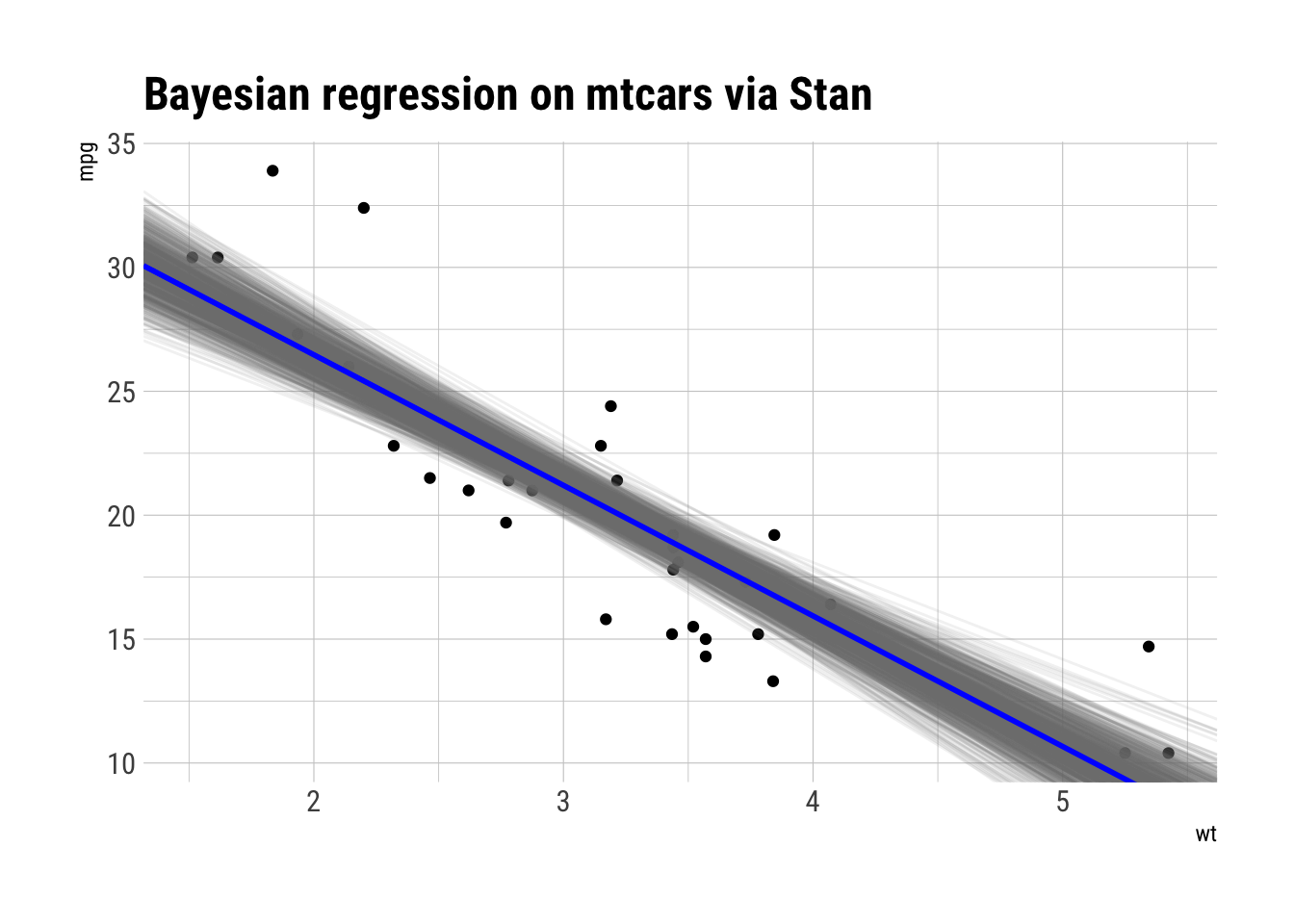Looks quite plausible for the data at hand.

## greta

Code for greta is very parallel to that of Stan, with the difference that code for a greta model is written entirely in R.

Input data is turned into a “greta array” object with as_data. Parameters are defined with distribution functions similar to Stan, though in greta they also produce greta arrays, albeit with not-yet-calculated data. There is no default uninformative prior in greta, so we’ll insert some broad but plausible values.

library(greta)

x <- as_data(mtcars$wt) y <- as_data(mtcars$mpg)

beta0 <- uniform(-100, 100)
beta1 <- uniform(-100, 100)
sigma <- uniform(0, 1000)

#> greta array (operation)
#>
#>       [,1]
#> [1,] 2.620
#> [2,] 2.875
#> [3,] 2.320
#> [4,] 3.215
#> [5,] 3.440
#> [6,] 3.460

beta0
#> greta array (variable following a uniform distribution)
#>
#>      [,1]
#> [1,]  ?

To combine our greta arrays into a model, we use ordinary arithmetic, leaving greta to keep track of array dimensions. We set our assumed distribution of the output by assigning to the distribution function in a fashion akin to names<-, but here setting our output distribution with our defined but uncalculated arrays.

Once everything is defined, we pass the yet-uncalculated objects we want to get out into the model function.

mu <- beta0 + beta1*x

distribution(y) <- normal(mu, sigma)

model_greta <- model(beta0, beta1, sigma)

None of the above objects individually look like much, but greta can use DiagrammeR to plot a beautiful dependency graph of the model:

plot(model_greta)To actually run the simulations, we pass the model to the mcmc function. Here I set the warmup and n_samples parameters to be parallel to Stan.

set.seed(47)

draws_greta <- mcmc(model_greta, warmup = 1000,  n_samples = 1000)

I am running this on my CPU, as the TensorFlow install is simpler. For such a small model, this is quite slow, taking about 2 minutes each for the warmup and sampling runs.2 It is supposed to scale well, though, so perhaps I am just running into overhead that will not grow or which would be much faster on more apt hardware. In any case, it does have a nice dynamic display showing the completed and remaining iterations and estimated time remaining, which seems pretty accurate, as progress bars go.

The resulting draws_greta object is a list of an array. It does not have a useful print method, but its mcmc.list class (from coda) does have nice methods for summary and diagnostic plots:

summary(draws_greta)
#>
#> Iterations = 1:1000
#> Thinning interval = 1
#> Number of chains = 1
#> Sample size per chain = 1000
#>
#> 1. Empirical mean and standard deviation for each variable,
#>    plus standard error of the mean:
#>
#>         Mean     SD Naive SE Time-series SE
#> beta0 37.148 2.1323  0.06743        0.10598
#> beta1 -5.313 0.6399  0.02024        0.03252
#> sigma  3.154 0.4468  0.01413        0.06356
#>
#> 2. Quantiles for each variable:
#>
#>         2.5%    25%    50%    75%  97.5%
#> beta0 33.160 35.717 37.109 38.610 41.293
#> beta1 -6.536 -5.729 -5.319 -4.888 -4.105
#> sigma  2.345  2.864  3.113  3.374  4.248

bayesplot::mcmc_intervals(draws_greta)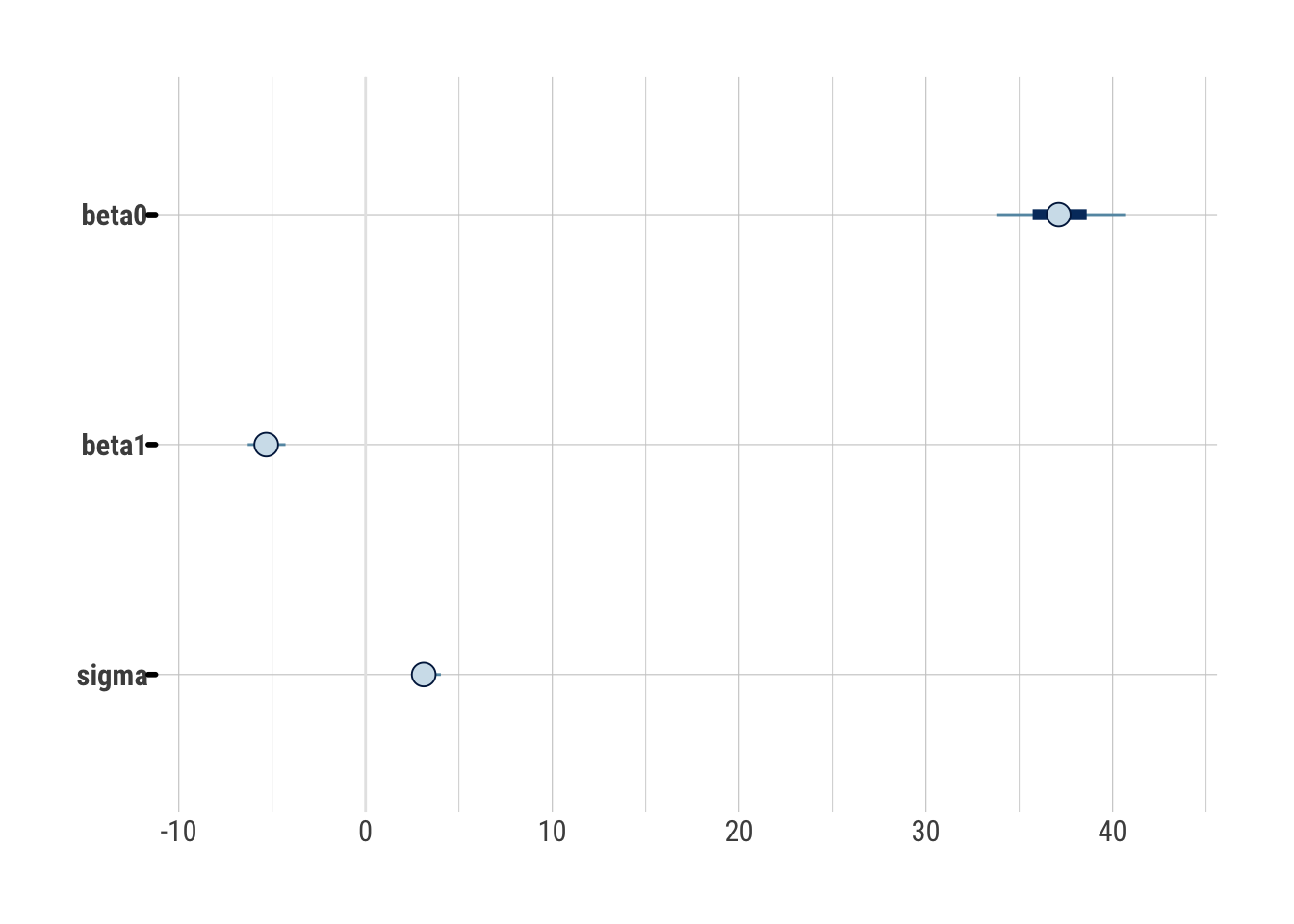bayesplot::mcmc_trace(draws_greta)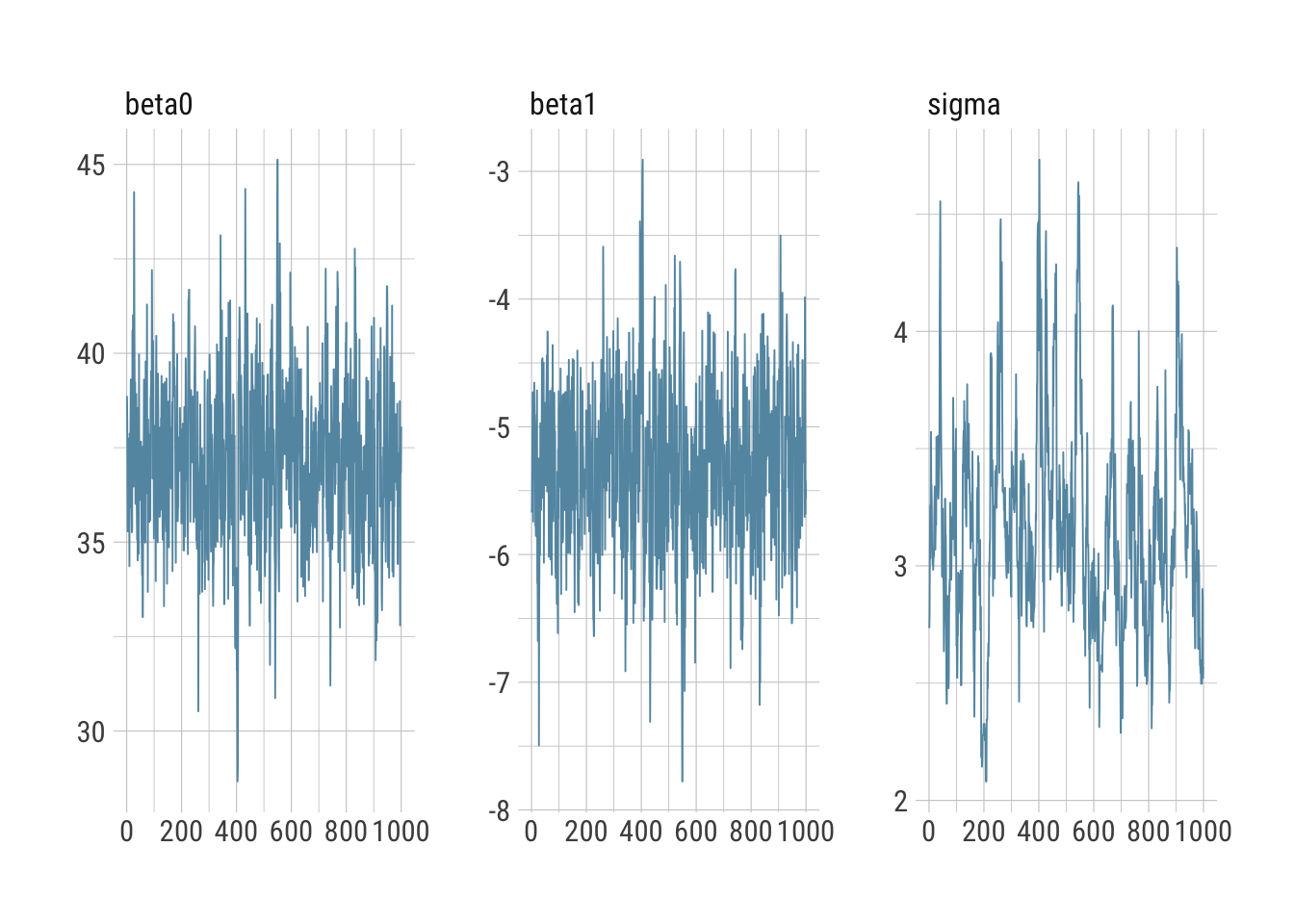Everything looks reasonable and comparable to Stan.

Let’s extract and plot the results:

draws_greta_df <- as.data.frame(draws_greta[])

ggplot(draws_greta_df, aes(beta0, beta1, color = sigma)) +
geom_point(alpha = 0.3) +
geom_density2d(color = "gray30") +
scale_color_viridis_c(option = "plasma") +
labs(title = "greta parameter space")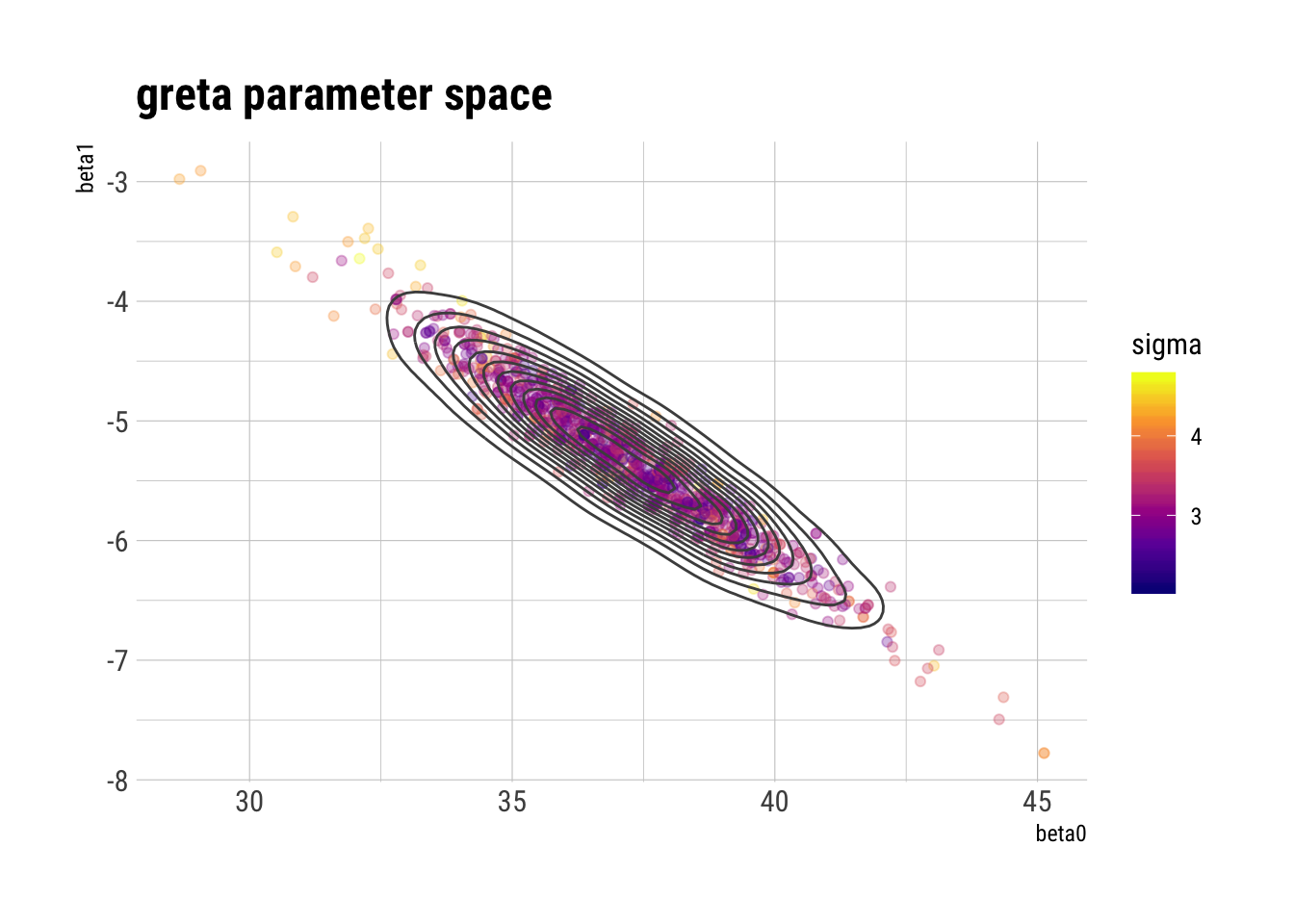ggplot(mtcars, aes(wt, mpg)) +
geom_point() +
geom_abline(aes(intercept = beta0, slope = beta1), draws_greta_df,
alpha = 0.1, color = "gray50") +
geom_abline(slope = mean(draws_greta_df$beta1), intercept = mean(draws_greta_df$beta0),
color = "blue", size = 1) +
labs(title = "Bayesian regression on mtcars via greta")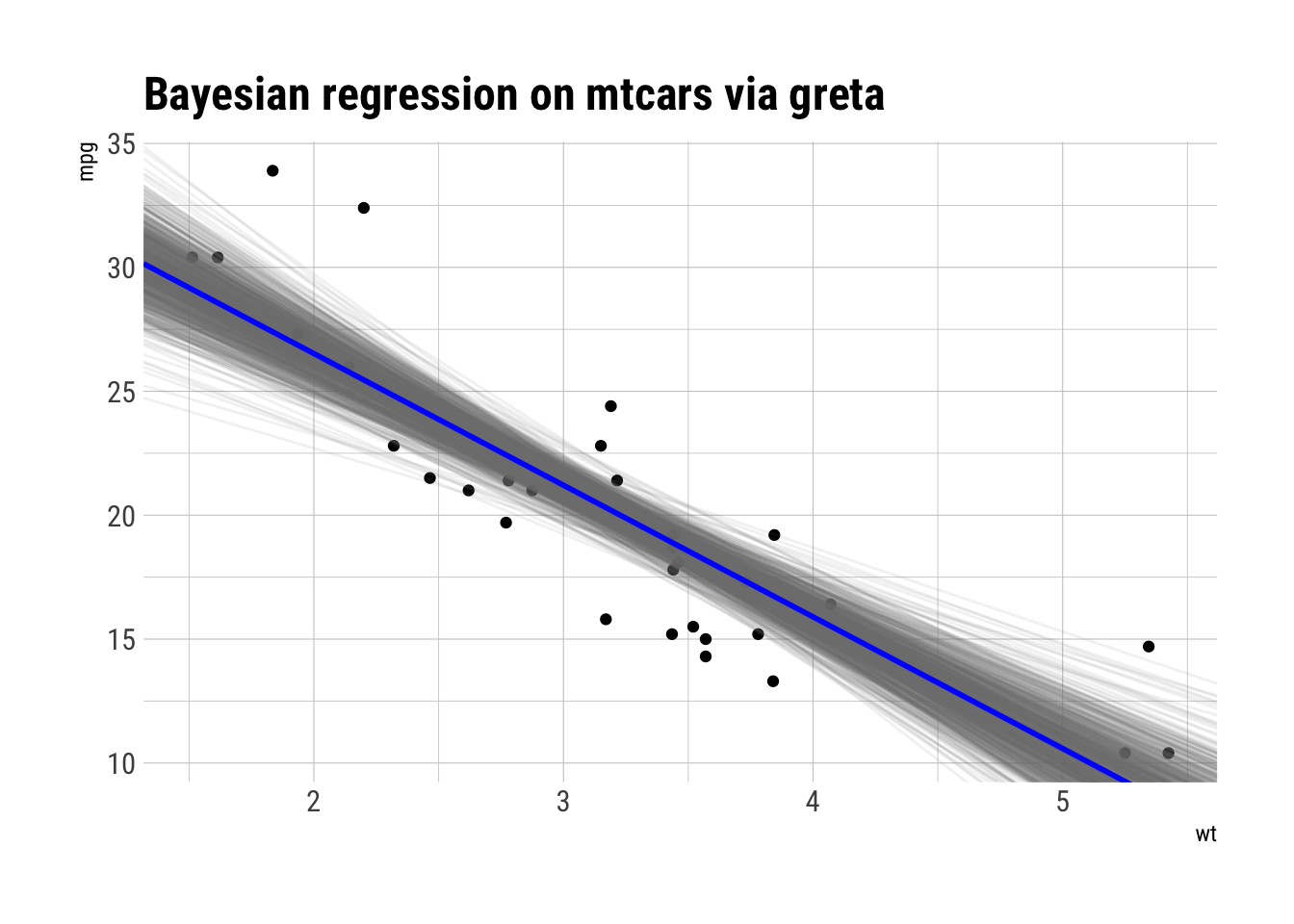Looks pretty similar to Stan.

## Reflections

### Stan

Stan is actually another language, although it is not particularly complicated or hard to learn. Since it is declarative imperative, it is easy to see what goes where in the model, but not so easy to figure out where bugs are when compilation fails. Error messages (aside from the annoying warnings) are pretty good, though.

Written in an RMarkdown chunk, Stan is really easy to integrate into a larger workflow without breaking stride. There is also the rstanarm package, which assembles up typical models into R functions. While this seems like it should be simpler, a quick attempt showed it to actually be somewhat more finicky, as the resulting functions have a lot of parameters and are fairly complicated compared to the smaller, simpler individual pieces of stan itself.

The biggest problem I ran into is that since Stan is another language, the documentation is not available with ?. In fact, it is a 637-page PDF. The PDF is in fact very well-written and organized, but still, it’s a PDF, which is annoying as it does not integrate into the web well. (I can’t put a link to the section on linear regression here.) Hopefully the documentation will eventually be better integrated into the otherwise-great Stan website. In an ideal world, it could be integrated into R’s help, too, though that would require some work.

### greta

greta avoids a lot of the issues of Stan. Documentation is available as usual. Intermediary products are easily viewable, and an individual problematic call will fail, making debugging simpler. It is not quite as easy to look at the code and quickly see the model structure, but the models plot method is a pretty solution to this problem.

In terms of organization, greta almost unavoidably leaves a lot of objects in the global environment with no inherent organization. The objects will not fit neatly in a data frame, so there is no natural organizational approach. Everything could (and perhaps should) be kept in a list or environment. A more robust approach would be to make a class with slots for the parts of the model and a suitable print method. While more work to build and apply, such an approach would allow quicker manipulations of multiple models without mess or confusion.

The biggest drawback in my short experiment is speed. I ran simulations on slightly larger (not “big”, in any sense) data, and it had no effect on run time, which seems to be purely a function of the number of iterations. I am not sure how it scales, but given that it was explicitly built for such a purpose, hopefully what I experienced is just overhead.

Overall, greta is a bit less mature than Stan, which has a bigger, funded team working on it. Given that greta leverages other projects that have such an infrastructure, it may not require such dedicated resources, but it does have room to grow yet. Hopefully it will!

### Bayesian simulation

For a relative beginner, both interfaces proved surprisingly simple and easily adaptable. I—as ever—have much yet to learn, but these frameworks will doubtlessly prove to be great assets with which to further explore the potential of Bayesian statistics.

1. h/t Daniel Lee. Thank you!

2. After upgrading to R 3.5.0 and reinstalling greta and TensorFlow, warmup and sampling take about 1 minute instead of 2. I’m not sure what caused the speedup, but it is wholly welcome!